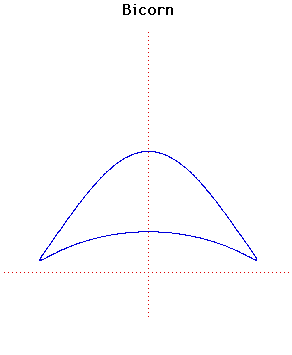# Curves

### BicornCartesian equation:
$y^{2}(a^{2} - x^{2}) = (x^{2} + 2ay - a^{2})^{2}$

### Description

The bicorn (also called the cocked-hat) is the name of a collection of quartic curves studied by Sylvester in 1864. The same curves were the studied by Cayley in 1867.

The particular bicorn given by Sylvester and Cayley is a different quartic from the one given here but this one, with a simpler formula, has essentially the same shape.

### Associated Curves

Definitions of the Associated curves